基于 matlab 的控制系统与仿真 3- 根轨迹、bode 图、Nyquist 图AXYZdong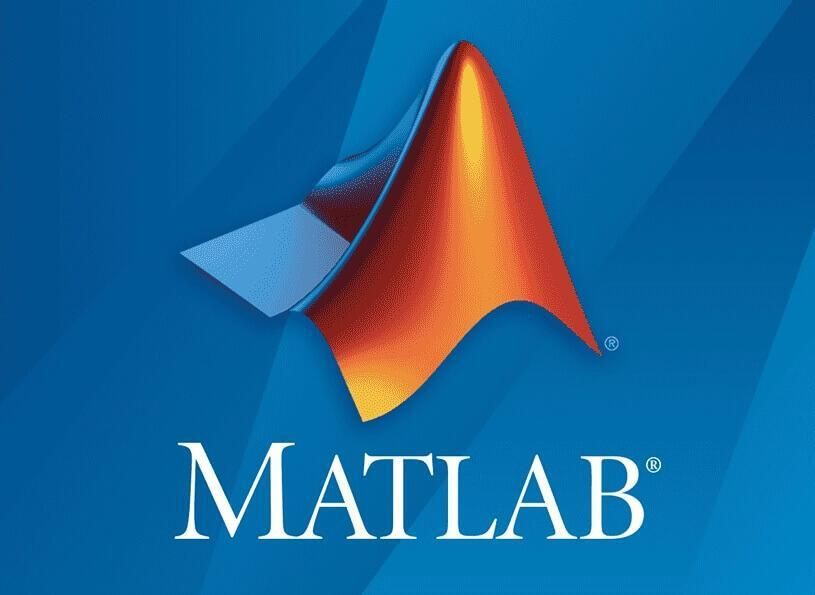Author：AXYZdong

根轨迹、bode 图、Nyquist 图

3.3 绘制以下传递函数模型的单位阶跃响应曲线

>>sys=tf([5 8],[1 4 6 3 3])
sys = 5 s + 8 ----------------------------- s^4 + 4 s^3 + 6 s^2 + 3 s + 3 Continuous-time transfer function.
>> step(sys)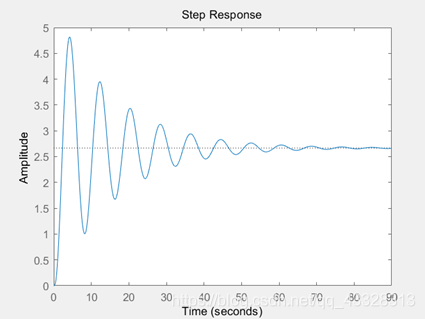3.7 绘制以下系统的根轨迹曲线

（1）

（2）

G1= tf(,conv([1 0],conv([1 2 2],[1 6 13])))
G1 = 1 ------------------------------------ s^5 + 8 s^4 + 27 s^3 + 38 s^2 + 26 s Continuous-time transfer function.
>> rlocus(G1)>> G2= tf([1 12],conv([1 1],conv([1 12 100],[1 10])))
G2 = s + 12 -------------------------------------- s^4 + 23 s^3 + 242 s^2 + 1220 s + 1000 Continuous-time transfer function.
>> rlocus(G2)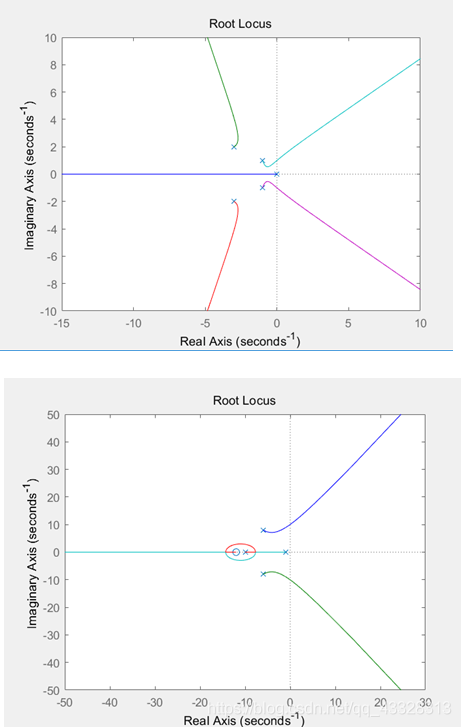3.8 已知反馈系统的开环传递函数为：

>> G3= tf([1 2 4],conv([1 0],conv([1 4],conv([1 6],[1 1.4 1]))))
G3 = s^2 + 2 s + 4 ----------------------------------------- s^5 + 11.4 s^4 + 39 s^3 + 43.6 s^2 + 24 s Continuous-time transfer function.
>> rlocus(G3)>> sgrid3.9 已知单位反馈控制系统的开环传递函数为：

（1）负反馈控制系统的根轨迹图；

（2）正反馈控制系统的根轨迹图；

>> G4= tf([1 2],conv([1 0],conv([1 4],conv([1 8],[1 2 5]))))
G4 = s + 2 --------------------------------------- s^5 + 14 s^4 + 61 s^3 + 124 s^2 + 160 s Continuous-time transfer function.
>> rlocus(G4)>> rlocus(-G4)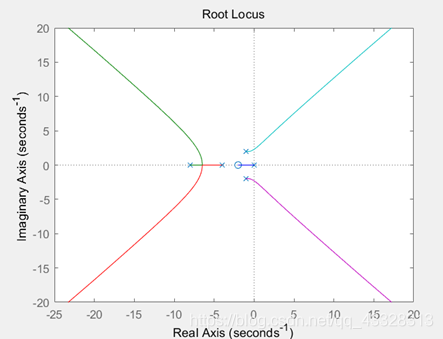▲ 负反馈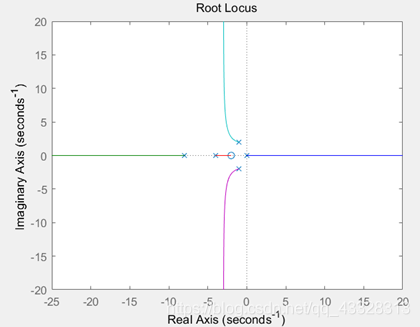▲ 正反馈

3.10 已知某控制系统的开环传递函数当 K=1.5 时，试绘制系统的开环频率特性曲线，并求出系统的幅值裕量与相位裕量。

G5=zpk([],[0 -1 -2],1.5)
G5 = 1.5 ------------- s (s+1) (s+2) Continuous-time zero/pole/gain model.
>> margin(G5)>> [Gm,Pm,Wcg,Wcp] = margin(G5)
Gm =
4.0000

Pm =
41.5340

Wcg =
1.4142

Wcp =
0.6118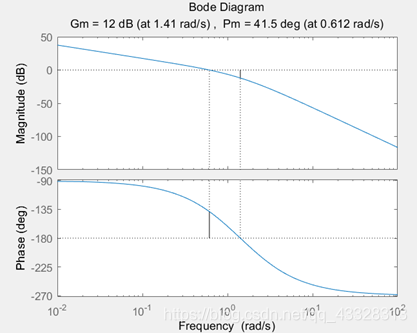3.11 已知一个典型的二阶环节传递函数为

wn=0.7;s=tf('s');n=[0.1,0.4,1.0,1.6,2.0];for i=nfigureG=wn^2/(s^2+2*i*wn*s+wn^2);bode(G);end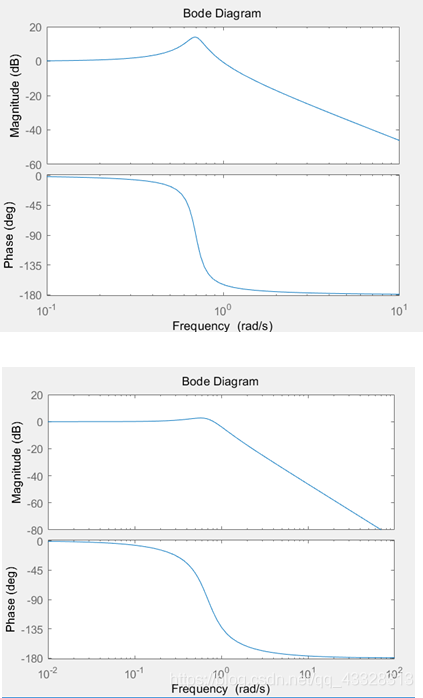3.13 已知系统开环传递函数为

>> G=zpk([-1],[-0.8-1.6*j,-0.8+1.6*j],3)
G = 3 (s+1) ------------------ (s^2 + 1.6s + 3.2) Continuous-time zero/pole/gain model.
>> nyquist(G)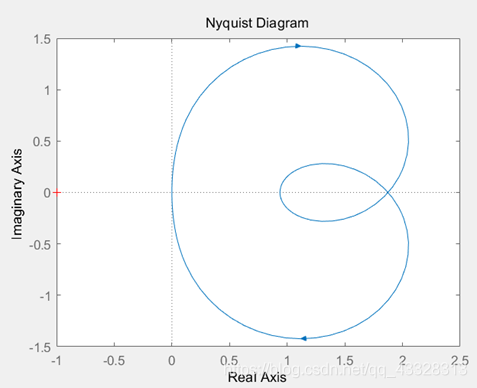3.14 已知二阶系统传递函数为：

n=[0.4,0.7,1.0,1.3];for i=nfigureG=tf([0 1],[1 2*i 1]);nyquist(G);end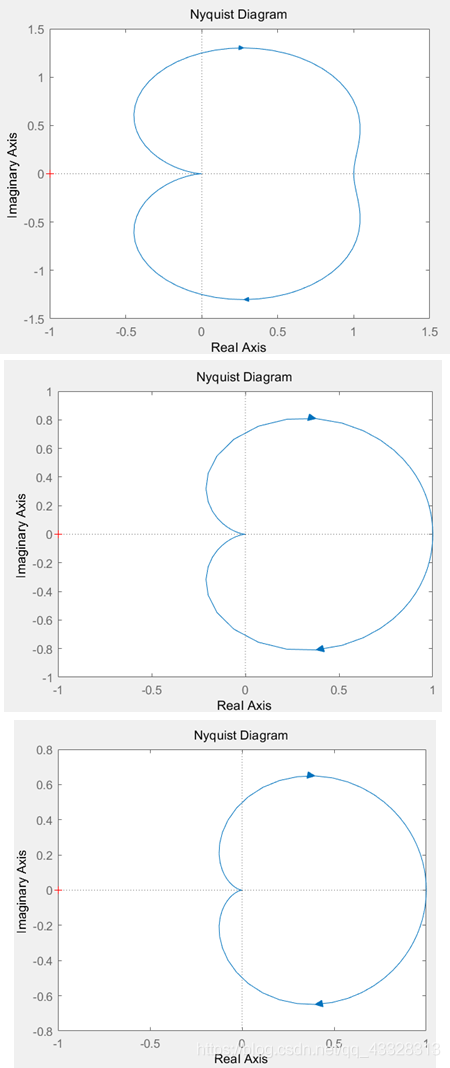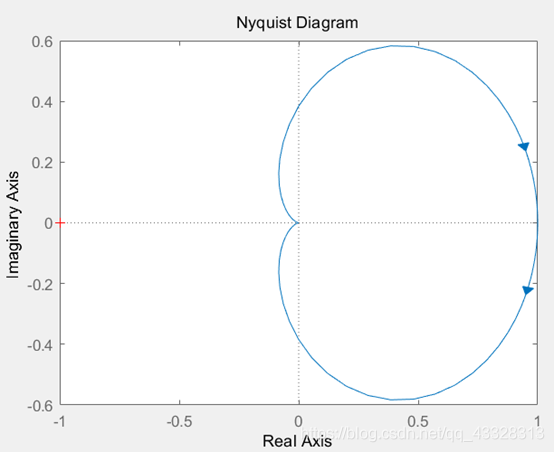评论